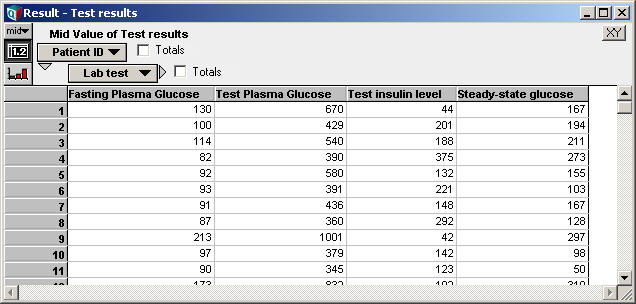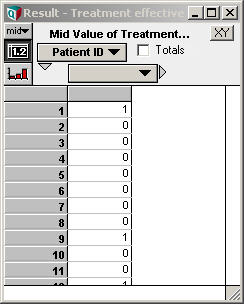# ProbitRegression

A probit model relates a continuous vector of dependent measurements to the probability of a binomial (i.e. 0,1-valued) outcome. In econometrics, this model is sometimes called the Harvard model. The ProbitRegression function infers the coefficients of the model from a data set, where each point in the training set is classified as 0 or 1.

Probit regression is very similar to LogisticRegression. Both are used to fit a binomial outcome based on a vector of continuous dependent quantities. They differ in their use of the link function. For the relationship, see these Wikipedia articles on the Wikipedia Generalized Linear Model and the probit model.

## ProbitRegression(Y, B, I, K, priorType, priorDev)

Given a set of data points, indexed by «I», with each point classified as 0,1 in the «Y» parameter, and a set of basis terms, «B», containing the dependent variables (where the vector of dependent variables is indexed by «K»), the ProbitRegression function finds and returns the set of coefficients for the probit model:

$\displaystyle{ Pr(Y=1|B=b) = \Theta^{-1}\left(\sum_k c_k b_k\right) }$

where $\displaystyle{ \Theta^{-1} }$ is the inverse cumulative normal distribution function.

The basis, «B», is a function of the dependent variables in your data. Each element along «K» of the basis vector may be an arbitrary, even non-linear, combination of the data in your data set. However, the number of terms in the basis should be kept small relative to the number of data point in your data set.

## Bayesian Prior

The procedure that fits a logistic regression to data is extremely susceptible to overfitting, and when overfitting occurs, you end up with a model that is too overconfident in its predictions. When logistic regression models overfit the data, they tend to output probabilities very close to 0% and 100% when presented with new data points, when such confidence is unwarranted. This problem is circumvented by using a Bayesian prior, which can also viewed as a penalty function for coefficients.

The «priorType» parameter allows you to select a Bayesian prior. The allowed values are

• 0: Maximum likelihood (i.e., no prior)
• 1: Exponential L1 prior
• 2: Normal L2 prior

The L1 and L2 priors impose a penalty for larger coefficient values, imposing a bias to keep coefficients small. Each imposes a prior probability distribution over the possible coefficient values, independently for each coefficient. The L1 prior takes the shape of an exponential curve, while the L2 prior takes the shape of a normal curve. There is no obvious reason for knowing whether an L1 or L2 would be better for your particular problem, and most likely that choice won't matter much.

The more important component of the prior is the «priorDev», which specifies the standard deviation of the prior -- i.e., how quickly the prior probability falls off. Larger values of «priorDev» correspond to a weaker prior. If you don't specify «priorDev», a guess is made by the function, which will typically be based on very little information. Cross-validation approaches can use the «priorDev» parameter to determine the best prior strength for a problem (see the Logistic Regression prior selection.ana example model in the Data Analysis folder in Analytica for an example).

Weaker priors will almost always result in a better fit on training data (and maximum likelihood should outperform any prior), but on a examples that don't appear in the training set, the performance can be quite a bit different. Typically, performance on new data will improve with weaker priors only up to a point, and then it will degrade and the prior is weakened further. The degradation is from the overfitting phenomena. These effects are observable in the following log-likelihood graph showing the performance on the training set and on a test set by ProbitRegression with an L2 prior. As you move to the right on the x-axis, the prior is getting weaker (this is the Breast Cancer data set, the graph is from the Logistic Regression prior selection.ana model).## Example

Suppose you want to predict the probability that a particular treatment for diabetes is effective given several lab test results. Data is collected for patients who have undergone the treatment, as follows, where the variable Test_results contains lab test data and Treatment_effective is set to 0 or 1 depending on whether the treatment was effective or not for that patient:Using the data directly as the regression basis, the logistic regression coefficients are computed using:

Variable c := ProbitRegression(Treatment_effective, Test_results, Patient_ID, Lab_test)

We can obtain the predicted probability for each patient in this testing set using:

Variable Prob_Effective := CumNormal(Sum(c*Test_results, Lab_Test))

If we have lab tests for a new patient, say New_Patient_Tests, in the form of a vector indexed by Lab_Test, we can predict the probability that treatment will be effective using:

CumNormal(Sum(c*New_patient_tests, Lab_test))

See the example for LogisticRegression for a further elaboration of this example, with additional notes on potential over-fitting problems that are common with this type of data analysis.

## History

ProbitRegression is new to Analytica 4.5.

In releases before 4.5, the Probit_Regression function is available to Analytica Optimizer users. The function here supersedes that function and does not require the Optimizer edition.